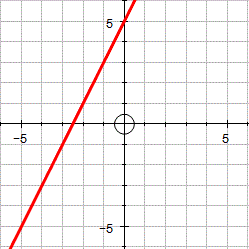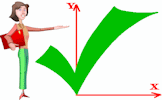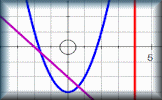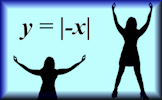# Graph Patterns :: A Coordinated Challenge

##### Level 1 :: Straight line (linear) graphsLevel 2 :: Quadratic graphsMore Graph Activities1

Can you figure out what equations we used to produce the pattern above? Type your answers below.

You don't have to wait until you have entered all of the equations before you click the Draw Graph button.
Sorry, your browser does not support this application. The followined:

This graph plotting challenge is intended to familiarise students with the equation of a straight line. There are five challenges each with between three and six straight lines in a pattern. In order to proceed to the next challenge the correct equation should be entered into the boxes provided.

The equation of any straight line can be written in the form:

## y = mx + c

where m is the gradient of the line and c is the y-coordinate of the point where the line crosses the y-axis (y-intercept).

The gradient of the line can be found by considering to points on the line where the exact coordinates can be determined. The rise is the vertical distance between these points and the run is the hirizontal distance. The gradient is the rise divided by the run (rise over run).

If the line slopes from bottom left to upper right the gradient is positive. If the line slopes from top left to bottom right the gradient is negative.For example the graph shown to the left has a gradient of 2 (for every one square across the line goes up two squares. Rise over run is 2 over 1 which is 2.) The y-intercept is 5 so the equation is:

## y = 2x + 5

A horizontal line with a y-intercept of three has equation:

## y = 3

A vertical line with an x-intercept of three has equation:

## x = 3

### Related activities

#### Straight Line Graphs10 straight line graph challenges for use with computer graph plotting software or a graphical display calculator.

Transum.org/go/?to=straight

#### Graph MatchMatch the equations with the images of the corresponding graphs. Linear, quadratic and more.

Transum.org/go/?to=graphmatch

#### Human GraphsStudents should be encouraged to stand up and make the shapes of the graphs with their arms.

Transum.org/go/?to=humangraphs

#### Plotting GraphsComplete a table of values then plot the corresponding points to create a linear, quadratic or reciprocal graph.

Transum.org/go/?to=plotting

The solutions to this and other Transum puzzles, exercises and activities are available in this space when you are signed in to your Transum subscription account. If you do not yet have an account and you are a teacher or parent you can apply for one here.